# Precalculus : Polynomial Functions

## Example Questions

1 2 6 7 8 9 10 11 12 14 Next →

### Example Question #1 : Solve Radical Equations And Inequalities

Solve this radical function: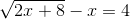Possible Answers:

None of these answers.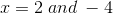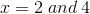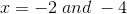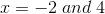Correct answer:Explanation:Add x to both sides: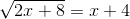Square both sides: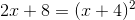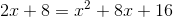Simplify: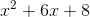Factor and set equal to zero: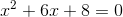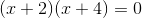### Example Question #9 : Radical Functions

Which of the following is and accurate graph of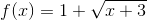?

Possible Answers: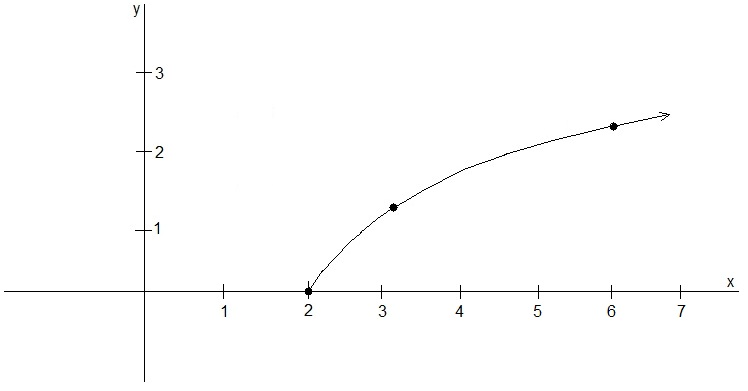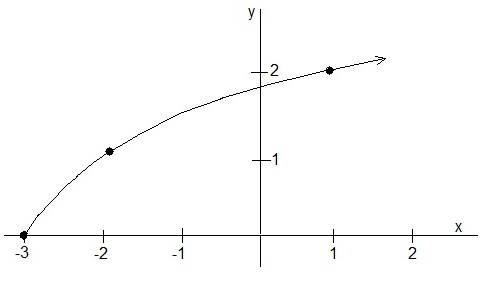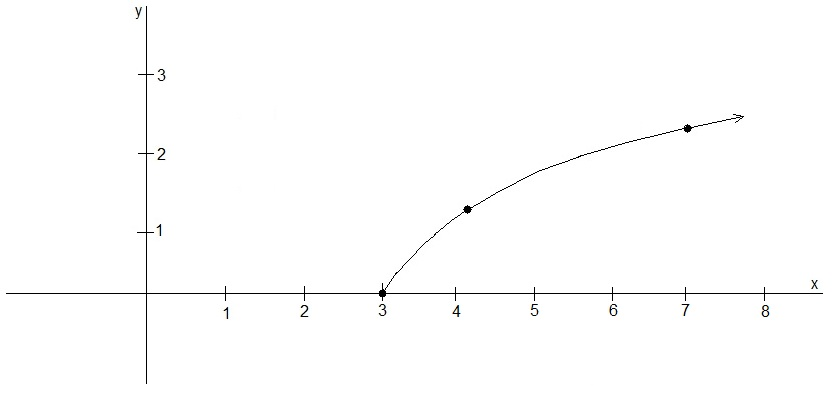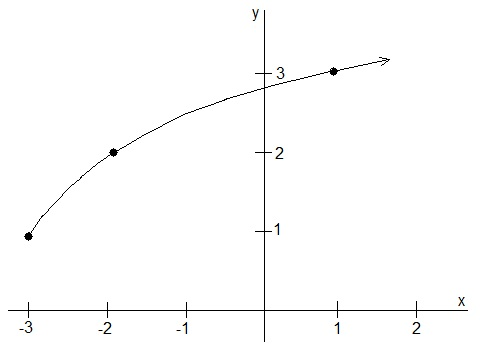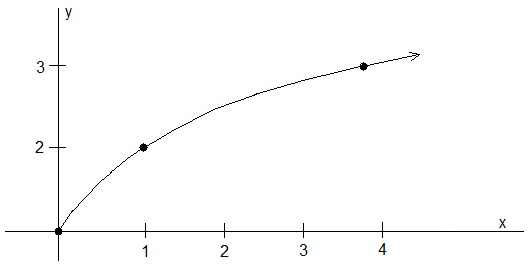Correct answer:Explanation:

Remember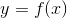, for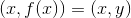.

Step 1, realize wherestarts: A) observe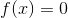never occurs, B) zero-out the radical component of;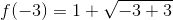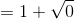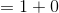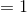C) The resulting point is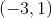.

Step 2, find simple points forafter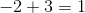, so use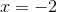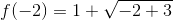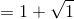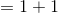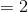The next resulting point;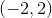.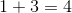, so use;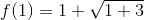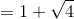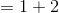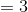The next resulting point;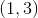.

Step 3, draw a curve through the considered points.

1 2 6 7 8 9 10 11 12 14 Next →

### All Precalculus Resources